# Top MCQs on Heap Data Strcuture with Answers

• Last Updated : 24 Aug, 2023

A Heap is a special Tree-based data structure in which the tree is a complete binary tree.
More on Heap Data Structure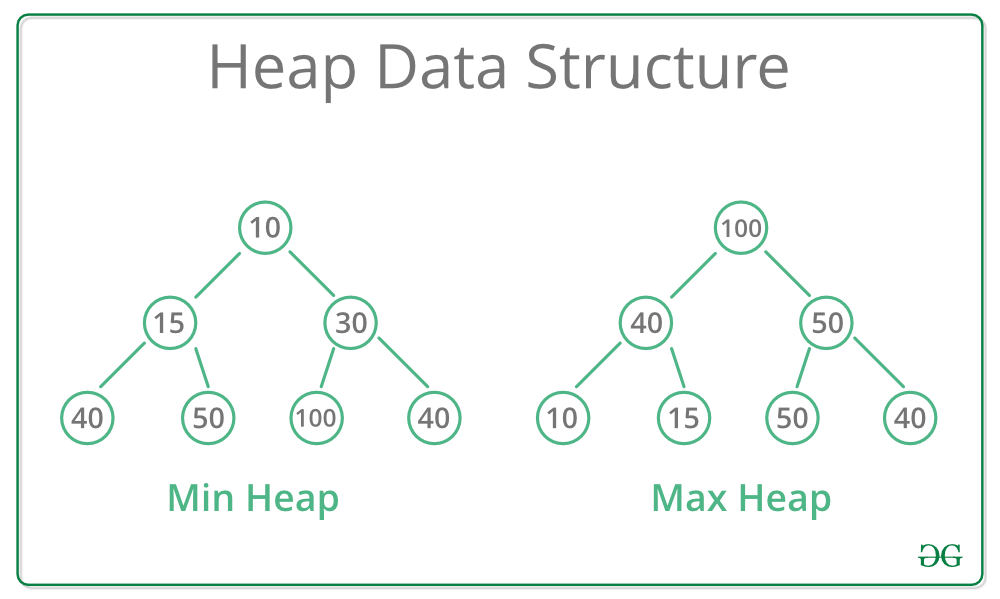Question 1

What is the time complexity of Build Heap operation. Build Heap is used to build a max(or min) binary heap from a given array. Build Heap is used in Heap Sort as a first step for sorting.O(nLogn)O(n^2)O(Logn)O(n)

Question 1-Explanation:

Following is algorithm for building a Heap of an input array A.

BUILD-HEAP(A)
heapsize := size(A);
for i := floor(heapsize/2) downto 1
do HEAPIFY(A, i);
end for
END

Although the worst-case complexity looks like O(N log N), upper bound of time complexity is O(n). See following links for the proof of time complexity. Time Complexity of building a heap

Hence Option(A) is the correct answer.

 Question 2

Suppose we are sorting an array of eight integers using heapsort, and we have just finished some heapify (either maxheapify or minheapify) operations. The array now looks like this: 16 14 15 10 12 27 28 How many heapify operations have been performed on root of heap?123 or 45 or 6

Question 2-Explanation:

In Heapsort, we first build a heap, then we do following operations till the heap size becomes 1. a) Swap the root with last element b) Call heapify for root c) reduce the heap size by 1. In this question, it is given that heapify has been called few times and we see that last two elements in given array are the 2 maximum elements in array. So situation is clear, it is maxheapify which has been called 2 times.

Hence Option(B) is the correct answer.

 Question 3

A max-heap is a heap where the value of each parent is greater than or equal to the values of its children. Which of the following is a max-heap? (GATE CS 2011) .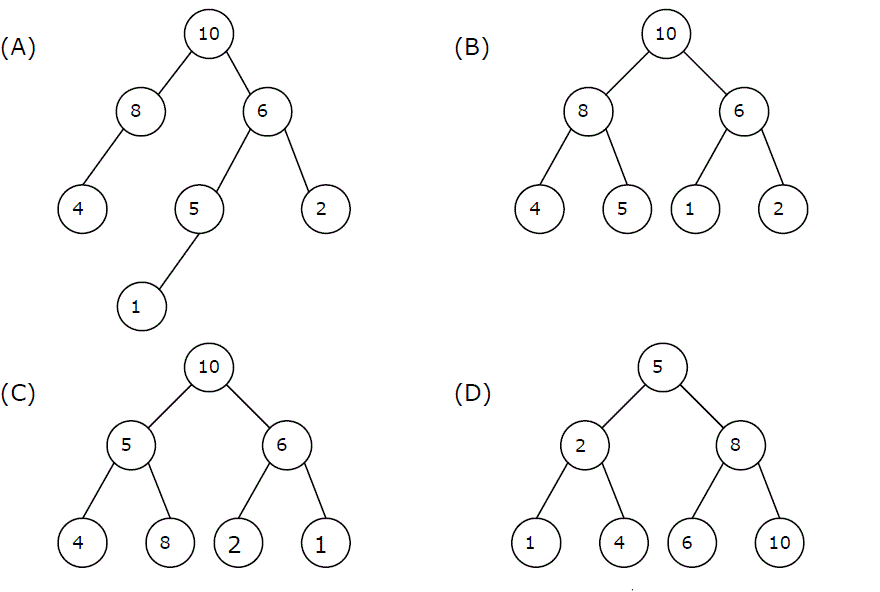ABCD

Question 3-Explanation:

(A) The provided tree is not a complete tree.
(B) The tree is a max-heap, as the root element is greater than all of its children elements.

• 10  > 8 and 6
• 8   > 4 and 5
• 6  > 1 and 2

(C) In the given tree, the Left root value 5 is smaller than 8, which is not following the constraints.
(D) In the given tree, the root value 5 is smaller than 8, which dissatisfies the condition.

Hence (B) is the correct Option.

 Question 4

A 3-ary max heap is like a binary max heap, but instead of 2 children, nodes have 3 children. A 3-ary heap can be represented by an array as follows: The root is stored in the first location, a, nodes in the next level, from left to right, is stored from a to a. The nodes from the second level of the tree from left to right are stored from a location onward. An item x can be inserted into a 3-ary heap containing n items by placing x in the location a[n] and pushing it up the tree to satisfy the heap property. Which one of the following is a valid sequence of elements in an array representing 3-ary max heap?1, 3, 5, 6, 8, 99, 6, 3, 1, 8, 59, 3, 6, 8, 5, 19, 5, 6, 8, 3, 1

Question 4-Explanation:

Following 3-ary Max Heap can be constructed from sequence given option (D)

                                      9
/  |   \
/     |     \
5       6      8
/  |
/    |
3      1
 Question 5

Suppose the elements 7, 2, 10 and 4 are inserted, in that order, into the valid 3- ary max heap found in the above question, Which one of the following is the sequence of items in the array representing the resultant heap?10, 7, 9, 8, 3, 1, 5, 2, 6, 410, 9, 8, 7, 6, 5, 4, 3, 2, 110, 9, 4, 5, 7, 6, 8, 2, 1, 310, 8, 6, 9, 7, 2, 3, 4, 1, 5

Question 5-Explanation:

After insertion of 7

                                          9
/   |   \
/     |     \
7       6       8
/ | \
/   |  \
3    1    5    

After insertion of 2

                                           9
/    |   \
/      |     \
7        6       8
/ | \       /
/   |  \     /
3    1    5  2

After insertion of 10

                                 10
/    |   \
/      |     \
7         9       8
/ | \       / |
/   |  \     /  |
3    1    5  2    6

After insertion of 4

                                 10
/   |   \
/     |     \
7        9       8
/ | \      / | \
/   |  \    /  |   \
3    1    5  2   6    4

Hence Option(A) is the correct answer.

 Question 6

Consider a binary max-heap implemented using an array. Which among the following arrays represents a binary max-heap?
(More than one option correct)25,12,16,8,10,13,1425,12,16,13,10,8,1425,14,16,13,10,8,1225,14,12,13,10,8,16

Question 6-Explanation:

A tree is max-heap if data at every node in the tree is greater than or equal to it’s children’ s data. In array representation of heap tree, a node at index i has its left child at index 2i + 1 and right child at index 2i + 2.

(A):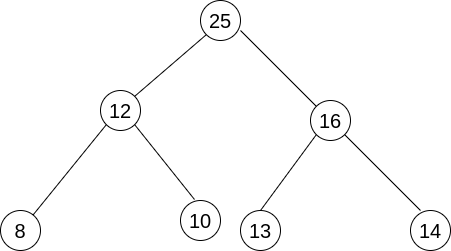(C) :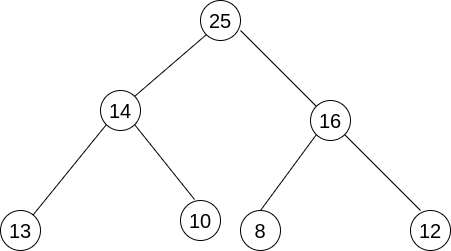So, the correct answer are A and C.

 Question 7

A min- Heap is a complete binary tree.TrueFalse

Question 7-Explanation:

A max-heap is a special Tree-based data structure in which the tree is a complete binary tree. A complete binary tree is a special type of binary tree where all the levels of the tree are filled completely except the lowest level nodes which are filled from as left as possible.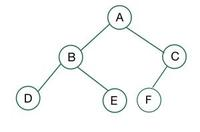Complete Binary Tree

Hence Option(A) is the correct answer.

 Question 8

We have a binary heap on n elements and wish to insert n more elements (not necessarily one after another) into this heap. The total time required for this is (A) theta(logn) (B) theta(n) (C) theta(nlogn) (D) theta(n^2)ABCD

Question 8-Explanation:

We can reduce the problem to build heap for 2n elements. Time taken for build heap is O(n).

Hence (B) is the correct answer.

 Question 9

In a min-heap with n elements with the smallest element at the root, the 7th smallest element can be found in time:theta(nlogn)theta(n)theta(logn)theta(1)

Question 9-Explanation:

For clarity, assume that there are no duplicates in Min-Heap and accessing heap elements below root is allowed.

The 7th smallest element must be in first 7 levels. Total number of nodes in any Binary Heap in first 7 levels is at most 1 + 2 + 4 + 8 + 16 + 32 + 64 which is a constant. Therefore we can always find 7th smallest element intime. If Min-Heap is allowed to have duplicates, then time complexity becomes Θ(Log n). Also, if Min-Heap doesn't allow directly accessing elements below root and supports only extract-min() operation, then also time complexity becomes Θ(Log n).

 Question 10

What are the necessary condition for a Tree to be a heap?the tree must be complete.Every Root value is greater or smaller than the children's value.Both A and BNone

Question 10-Explanation:

A Heap is a special Tree-based data structure in which the tree is a complete binary tree.

### Types of Heap Data Structure

Generally, Heaps can be of two types:

1. Max-Heap: In a Max-Heap the key present at the root node must be greatest among the keys present at all of its children. The same property must be recursively true for all sub-trees in that Binary Tree.
2. Min-Heap: In a Min-Heap the key present at the root node must be minimum among the keys present at all of its children. The same property must be recursively true for all sub-trees in that Binary Tree.Hence Option(C) is the correct answer.

There are 30 questions to complete.